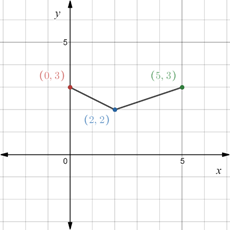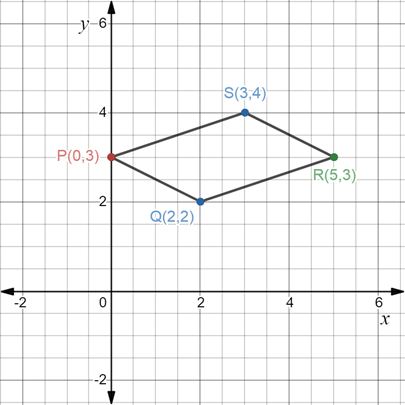# To graph : The parallelogram PQRS where coordinates of the point P, Q and R are P ( 0 , 3 ) , Q ( 2 , 2 ) , R ( 5 , 3 ) . Also compute the coordinate of the point Q .### Precalculus: Mathematics for Calcu...

6th Edition
Stewart + 5 others
Publisher: Cengage Learning
ISBN: 9780840068071### Precalculus: Mathematics for Calcu...

6th Edition
Stewart + 5 others
Publisher: Cengage Learning
ISBN: 9780840068071

#### Solutions

Chapter 1.8, Problem 119E
To determine

## To graph: The parallelogram PQRS where coordinates of the point P, Q and R are P(0,3),Q(2,2),R(5,3) . Also compute the coordinate of the point Q .

Expert Solution

### Explanation of Solution

Given information:

PQRS is a parallelogram and coordinates of three points are P(0,3),Q(2,2),R(5,3) .

Graph:

Plot the points P(0,3),Q(2,2),R(5,3) on the coordinate plane.Let the coordinates of the point S be (x,y) .

It is known that diagonals of the parallelogram bisect each.

The parallelogram has two diagonals PR and QS .

Let M(a,b) be the midpoint of the diagonal joined by the line segment PR .

Recall that the midpoint of the segment that joins two points A=(x1,y1) and B=(x2,y2) in the Cartesian plane is denoted by (x1+x22,y1+y22) .

Now, M(a,b) is the mid-point of the line segment joined by the points P(0,3) and R(5,3) .

Therefore,

(0+52,3+32)=(a,b)(52,62)=(a,b)(52,3)=(a,b)

Therefore, M(52,3) is the mid-point of the line segment joined by the points P(0,3) and R(5,3) .

Also, M(52,3) is the mid-point of the line segment joined by the points Q(2,2) and S(x,y) .

(2+x2,2+y2)=(52,3)

Equate the x -coordinate of each bracket,

2+x2=522+x=5x=3

Equate the y -coordinate of each bracket,

2+y2=32+y=6y=4

Therefore, coordinates of the point S are S(3,4) .

Now, plot the points P(0,3),Q(2,2),R(5,3) and S(3,4) on the coordinate plane.Interpretation:

The parallelogram PQRS so formed above, has diagonals that bisect each other. Opposites sides are congruent. Opposite angles are congruent. Consecutive angles are supplementary that is adds to 180° .

### Have a homework question?

Subscribe to bartleby learn! Ask subject matter experts 30 homework questions each month. Plus, you’ll have access to millions of step-by-step textbook answers!